Question

# The critical angle for total internal reflection for diamond (n=2.42) surrounded by water (n=1.33) is approximately...

The critical angle for total internal reflection for diamond (n=2.42) surrounded by water (n=1.33) is approximately what? Answer: 33 degrees.

The possible choices were:

(a) 24 degrees.

(b) 33 degrees.

(c) 49 degrees.

(d) 63 degrees.

(e) 90 degrees.

feel free to drop comment if you have any doubt.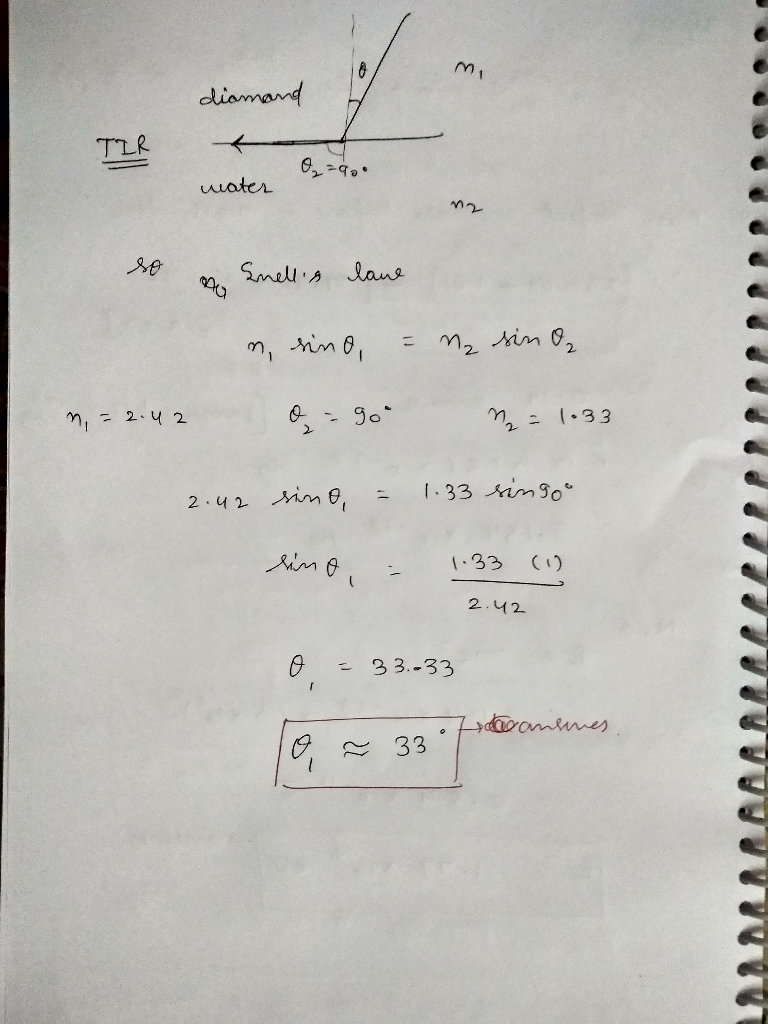#### Earn Coins

Coins can be redeemed for fabulous gifts.

Similar Homework Help Questions
• ### Calculate the critical angle of total internal reflection (TIR) for the interface between water (n=1.33) and...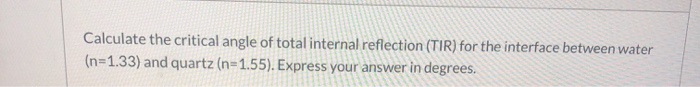Calculate the critical angle of total internal reflection (TIR) for the interface between water (n=1.33) and quartz (n=1.55). Express your answer in degrees.

• ### A fiber optic cable is submerged underwater. What is the critical angle of total internal reflection...

A fiber optic cable is submerged underwater. What is the critical angle of total internal reflection if the index of refraction of the cable and water is n cable=2.15 and n water=1.33? options in degrees: 15.12, 42.79, 38.21, 61.33

• ### What is the angle of total internal reflection (critical angle) for a glass-to-water interface? Assume a...

What is the angle of total internal reflection (critical angle) for a glass-to-water interface? Assume a refractive index of 1.72 for the glass and 1.33 for the water.

• ### When light passes through a certain medium that is surrounded by air, the critical angle for...

When light passes through a certain medium that is surrounded by air, the critical angle for total internal reflection is 45°. If that medium is submerged in water (n = 1.33), what is the new critical angle?

• ### please show work the ray of light in air hits the surface of water (n=1.33) at...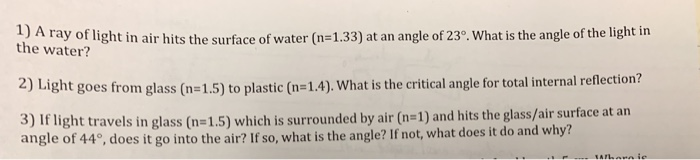please show work the ray of light in air hits the surface of water (n=1.33) at an angle of 23° what is the angle of the light in 2) Light goes from glass (n-1.5) to plastic (n-1.4). What is the critical angle for total internal reflection? 3) If light travels in glass (n-1.5) which is surrounded by air (n- 1) and hits the glass/air surface at an angle of 44, does it go into the air? If so, what is...

• ### 2. A 50% solution of glucose in water has a critical angle for total internal reflection...

2. A 50% solution of glucose in water has a critical angle for total internal reflection of 45 degrees. Suppose a beam of light passes from the air down into such a solution at an incident angle of 45 degrees. What angle will the beam make in the solution?

• ### The critical angle for total internal reflection when going from one type of glass to water...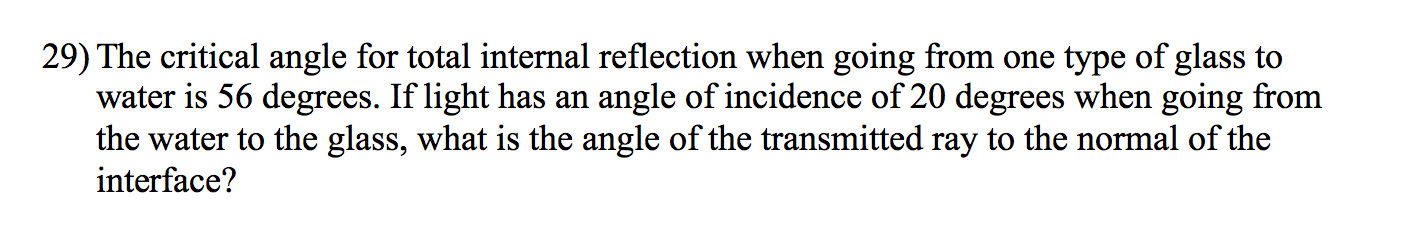The critical angle for total internal reflection when going from one type of glass to water is 56 degrees. If light has an angle of incidence of 20 degrees when going from the water to the glass, what is the angle of the transmitted ray to the normal of the interface?

• ### Snell's Law and Total Internal Reflection A core of material is surrounded by another material. What...

Snell's Law and Total Internal Reflection A core of material is surrounded by another material. What is the ratio of inner index to outer if the critical angle is to be 37 degrees.

• ### What is the critical angle for light incident on water water = 1.33 from air nair...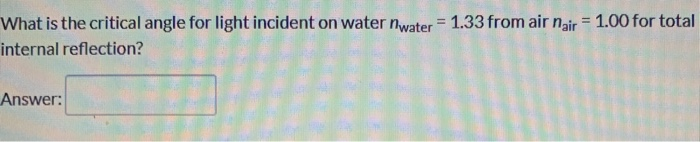What is the critical angle for light incident on water water = 1.33 from air nair = 1.00 for total internal reflection? Answer:

• ### What is the critical angle for total internal reflection for a material with an index of...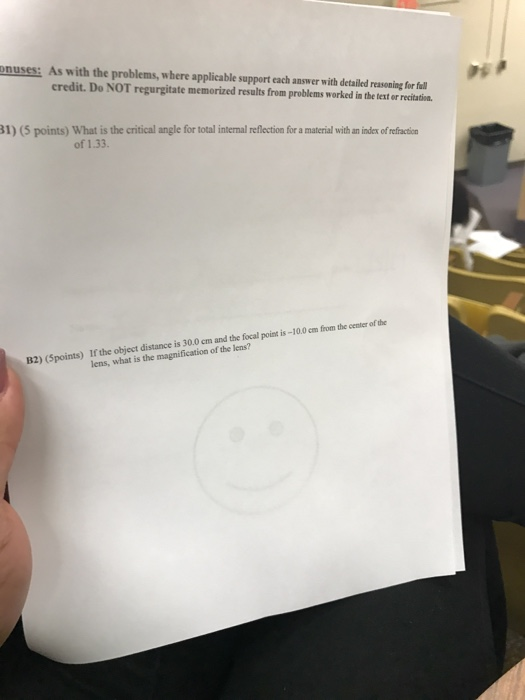What is the critical angle for total internal reflection for a material with an index of refraction of 1.33. If the object distance is 30.0 cm and the focal point is -10.0 cm from the center of the lens, what is the magnification of the lens?